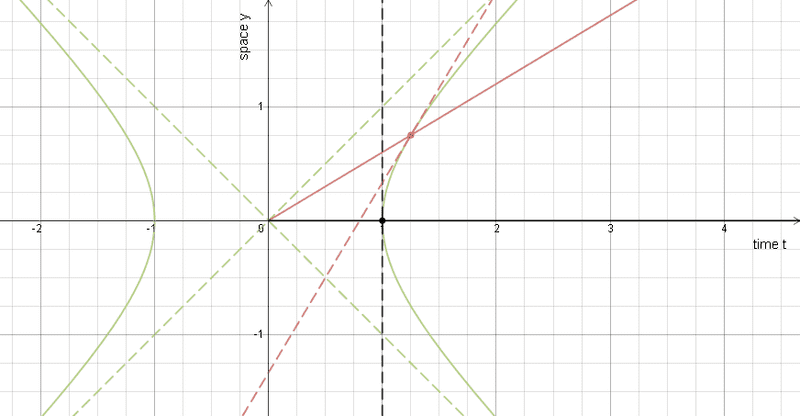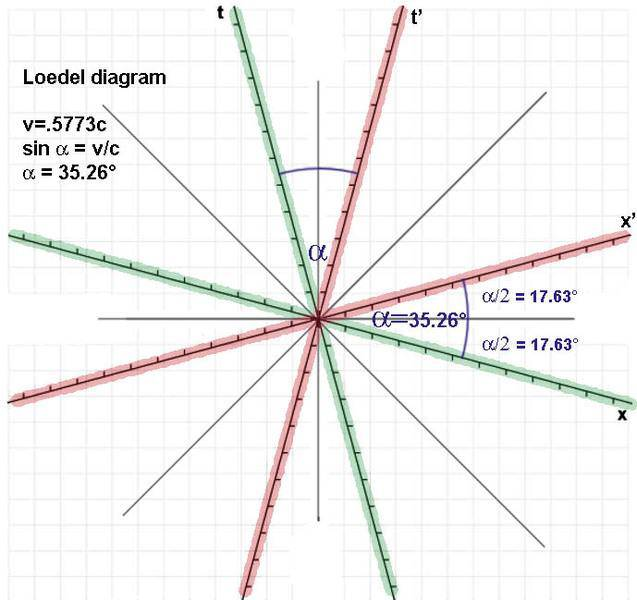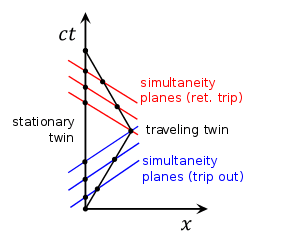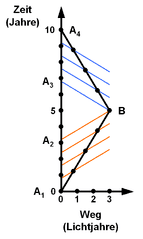# Minkowski diagrams without scales on axes

• I
Hi.

I have seen quite a lot of demonstrations of time dilation and length contraction that used standard Minkowski diagrams WITHOUT any scales on the axes at all. If I understand them correctly they seem to directly compare lengths, which would imply (I think) that the scaling on the ##ct/x## and ##ct'/x'## axes is the same.

But it is not. If in a standard Minkowski diagram the unit length on the axes of ##ct## and ##x## is given as ##U##, the unit length on the axes of ##ct'## and ##x'## is
$$U'=U\cdot \sqrt{\frac{1+\beta^2}{1-\beta^2}} .$$
I can see how Minkowski diagrams without scales can be used to talk about simultaneity or the temporal order of events in different inertial systems, but can they illustrate time dilation and length contraction where the actual length of intervals in time or space need to be compared? I doubt it because in the derivation of the angle between the ##ct## and ##ct'## axes one looks at all events with ##x'=0## and uses the Lorentz transform
$$0=x'=\gamma\cdot (x-vt)\Rightarrow \tan\alpha=\frac{x}{ct}=\frac{v}{c}$$
and analogously for the angle between the ##x## and ##x'## axes, which turns out to be ##\alpha## as well. The Lorentz factor ##\gamma## drops out in the derivation of the angle, so why should it turn up again in a graphical derivation of time dilation or length contraction?

Am I right about this and is it merely a coincidence that those demonstrations explain time dilation and length contraction qualitatively correct?

Second question:
Is there a way to construct ##U'## geometrically in a Minkowski diagram with given angle? I know there are symmetric Minkowski (Loedel) diagrams that cirumvent this problem, but they are far less common than the standard diagrams where the ##ct/x## system is at rest.

Dale
Mentor
2021 Award
I have seen quite a lot of demonstrations of time dilation and length contraction that used standard Minkowski diagrams WITHOUT any scales on the axes at all
Can you link to one such demonstration as a concrete example?

I can: http://www.ep5.ruhr-uni-bochum.de/cz-physik1/Vorlesung26.pdf [Broken]
Slides 5 to 7. It's in German, but I think the diagrams are self-explanatory.

Or Wikibooks.

Also, Google Image Search for 'minkowski diagram time dilation' or 'minkowski diagram length contraction' finds quite a lot of diagrams without scales, but I haven't checked many of them.

Last edited by a moderator:
Dale
Mentor
2021 Award
I can: http://www.ep5.ruhr-uni-bochum.de/cz-physik1/Vorlesung26.pdf [Broken]
Slides 5 to 7. It's in German, but I think the diagrams are self-explanatory
I agree with your criticism for this diagram.

Last edited by a moderator:
•greypilgrim
vanhees71
Gold Member
2021 Award
Yep, it's just wrong! Forget these lecture notes :-(. That's the reason, why I don't like Minkowski diagrams very much. It's more work to learn to draw and read them correctly than to do the analytical calculations!

•MikeLizzi, greypilgrim and Dale
Orodruin
Staff Emeritus
Homework Helper
Gold Member
2021 Award
Second question:
Is there a way to construct ##U'## geometrically in a Minkowski diagram with given angle? I know there are symmetric Minkowski (Loedel) diagrams that cirumvent this problem, but they are far less common than the standard diagrams where the ##ct/x## system is at rest.

Yes, you can easily normalise arbitrary axes in a Minkowski diagram by drawing the hyperbolae ##x^2 - c^2 t^2 = \pm 1##. Just as you could normalise rotated axes in Euclidean space by drawing the circle ##x^2 + y^2 = 1##.

•greypilgrim and vanhees71
Orodruin
Staff Emeritus
Homework Helper
Gold Member
2021 Award
•jim mcnamara and greypilgrim
Yes, you can easily normalise arbitrary axes in a Minkowski diagram by drawing the hyperbolae x2−c2t2=±1x^2 - c^2 t^2 = \pm 1. Just as you could normalise rotated axes in Euclidean space by drawing the circle x2+y2=1x^2 + y^2 = 1.
Thank you. Well I guess in most applications it's hugely impractical to really construct those hyperbolae geometrically, that's why I'm particularly thankful for
Also, see Ibix's interactive Minkowski diagram tool Interactive Minkowski diagrams
as I've been looking for an easy way to draw empty Minkowski diagrams.

robphy
Homework Helper
Gold Member
In old related threads ( here and here ), I posted my diagrammer https://www.desmos.com/calculator/ti58l2sair
which could be modified to draw fancier diagrams.There is a way to do quantitatively correct diagrams without explicitly using hyperbolas or detailed calculations.
I'll write more when I have the time.

Correct me if I'm wrong but isn't the ct' axis going to be arctan(c/u) from the ct axis?

robphy
Homework Helper
Gold Member
I think you mean the angle arctan(u/c) in degrees. When u=0, the angle will be zero degrees.
While degrees are useful for drawing with typical Euclidean tools, the angle between timelike directions is more naturally described by the rapidity (arc length along a unit hyperbola) as arctanh(u/c).

•Battlemage!
Orodruin
Staff Emeritus
Homework Helper
Gold Member
2021 Award
arc length along a unit hyperbola
Also, just to clarify, this is the arc length with the Minkowski metric.

There is a way to do quantitatively correct diagrams without explicitly using hyperbolas or detailed calculations.
I'll write more when I have the time.

Looking forward to this, I can't imagine how this could be possible.

•vanhees71
Yep, it's just wrong! Forget these lecture notes :-(. That's the reason, why I don't like Minkowski diagrams very much. It's more work to learn to draw and read them correctly than to do the analytical calculations!
Yes, but the spacetime diagram shows you how the different 3D worlds of simultanous events evolve though 4Dspacetime. You never get such a valuable 4D insight with only analytical calculations.

Hi.

I know there are symmetric Minkowski (Loedel) diagrams that cirumvent this problem, but they are far less common than the standard diagrams where the ##ct/x## system is at rest.
That's no reason for not using them. If you need only two frames to deal with (as is mostly the case in exercises) there is no reason to use a Minkowski diagram. In a Loedel diagram it's a lot easier to consider each frame at rest because none of both frames has orthogonal axes. A layman often never gets that far with a Minkowski diagram where the orthogonal x,t frame is often erroneously treated as a preferred 'at rest' frame.

It's very easy to draw a two frame spacetime diagram with identical unit lengths. All you need is calculating the angle. Then make your own unit length as you like. But remember: the unit length is only valid for the two frames (don't be tempted using it on the vertical and horizontal grey orthogonal lines).
An Loedel example for v=.5773c:vanhees71
Gold Member
2021 Award
That's a clever trick, using the light-cone coordinates in "invariant reference frame". The only trouble with this is that I have to get used to another type of space-time diagrams, which I don't find very intuitive anyway (no matter whether you have Minkowski or whatever other diagrams). For me, formulae are the intuitive picture ;-)).

robphy
Homework Helper
Gold Member
There is a way to do quantitatively correct diagrams without explicitly using hyperbolas or detailed calculations.
I'll write more when I have the time.

Looking forward to this, I can't imagine how this could be possible.

Here it is:
A new entry for Physics Forums Insights has been posted
https://www.physicsforums.com/insights/spacetime-diagrams-light-clocks/

•greypilgrim
The representation of the twin paradox on the English Wikipedia vs. the German one:I'm not sure if the black dots on the axes of the English image are supposed to be a scale (which would be wrong), but I think it's highly misleading.

vanhees71